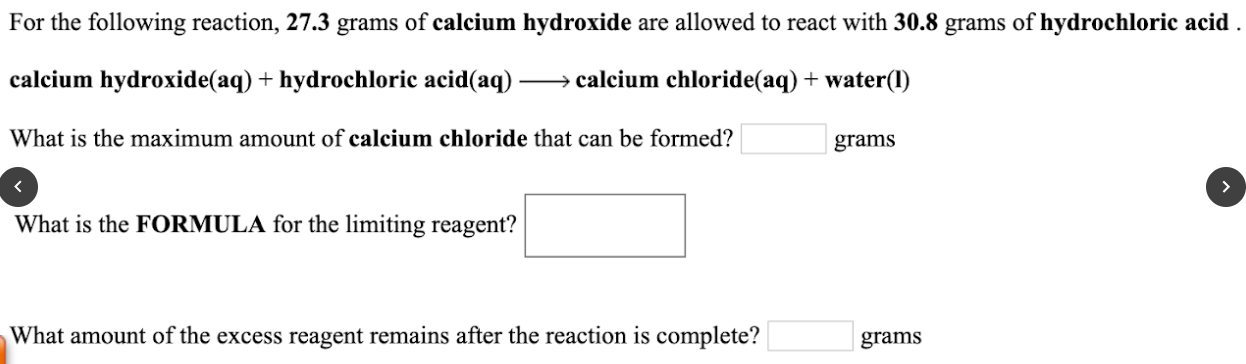# For the following reaction, 27.3 grams of calcium hydroxide are allowed to react with 30.8 grams of hydrochloric acid. calcium hydroxide(aq) + hydrochloric acid(aq) → calcium chloride(aq) + water(I) What is the maximum amount of calcium chloride that can be formed? ____ grams What is the FORMULA for the limiting reagent? What amount of the excess reagent remains after the reaction is complete? ____ grams